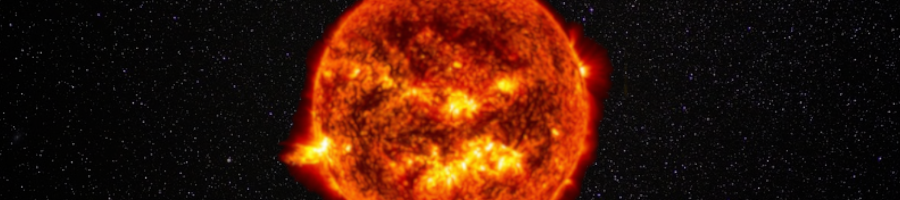ULYSSES

Ulysses HISCALE Data Analysis Handbook

## Appendix 10. Effect of Backscattered Electrons on the Geometric Factors of the LEMS30 Telescope (Hong MS Thesis)

### A10.10 Appendix - Trajectories of the Escaped Electrons

The following figures show the escaped electron trajectories of the backscattered electrons which start from the center of the detector for the different energies. (Those trajectories of escaped electrons without any impacts with the wall of the telescope are not shown here.) Figures A10-17 and A10-18 show the results when Radzimski's backscattering coefficients are used. Figure A10-17 shows the view angle of q = 0º and f = 90º. Figure A10-18 shows the view angle of q = -20º and f = 80º. Figures A10-19 and A10-20 are the trajectories for Neubert's backscattering coefficients.

Figure A10-17a View angle of q = 0º and f = 90º. Radzimski's backscattering coefficients are used.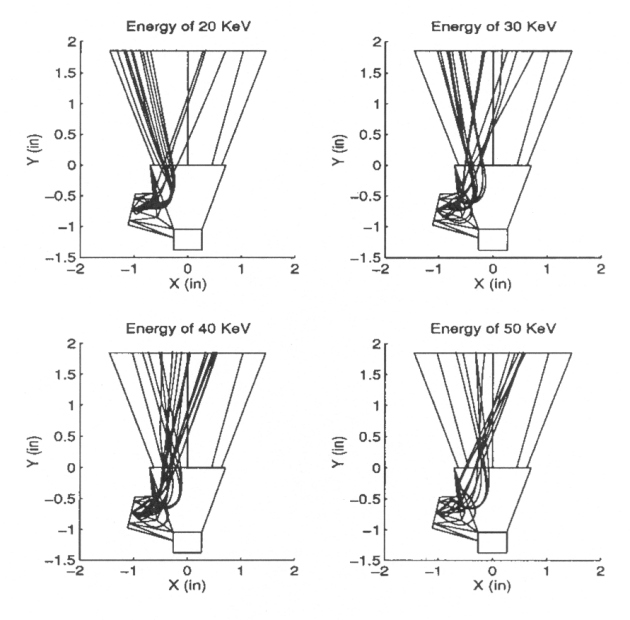Figure A10-17b View angle of q = 0º and f = 90º. Radzimski's backscattering coefficients are used.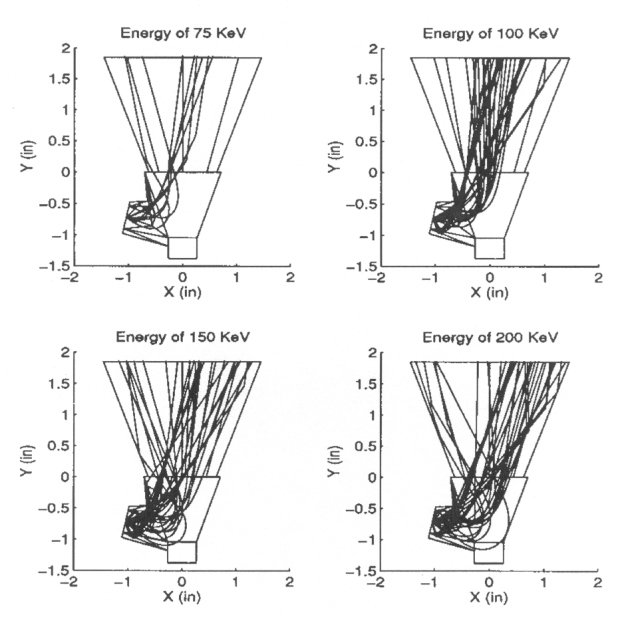Figure A10-17c View angle of q = 0º and f = 90º. Radzimski's backscattering coefficients are used.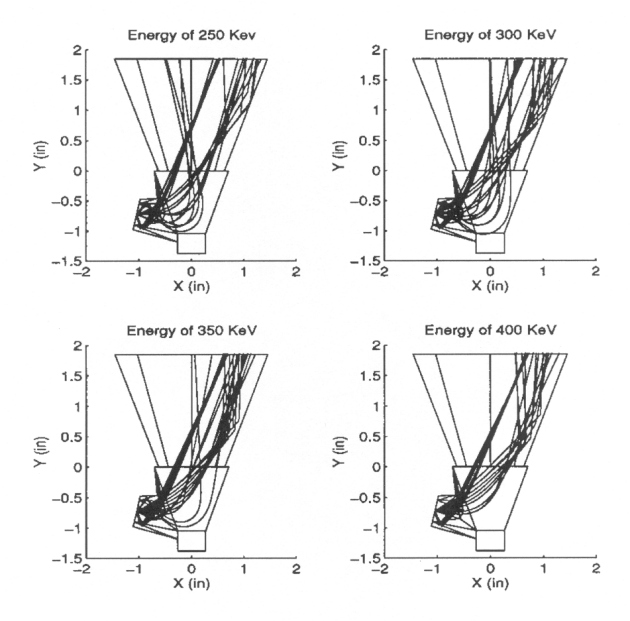Figure A10-18a View angle of q = -20º and f = 80º. Radzimski's backscattering coefficients are used.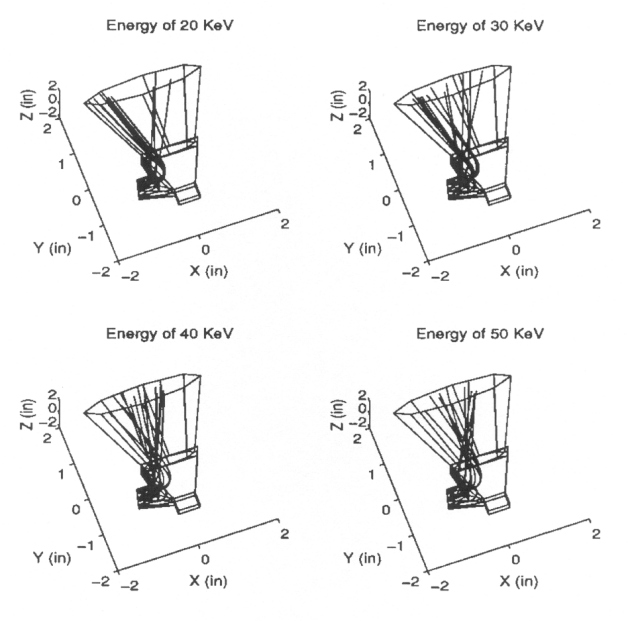Figure A10-18b View angle of q = -20º and f = 80º. Radzimski's backscattering coefficients are used.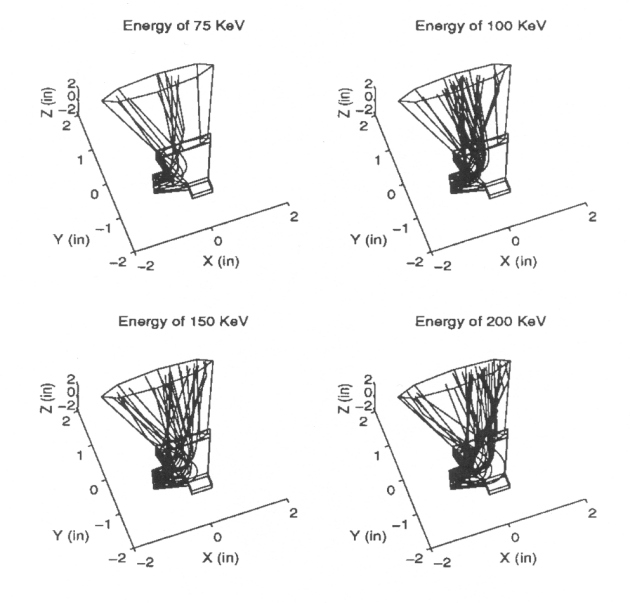Figure A10-18c View angle of q = -20º and f = 80º. Radzimski's backscattering coefficients are used.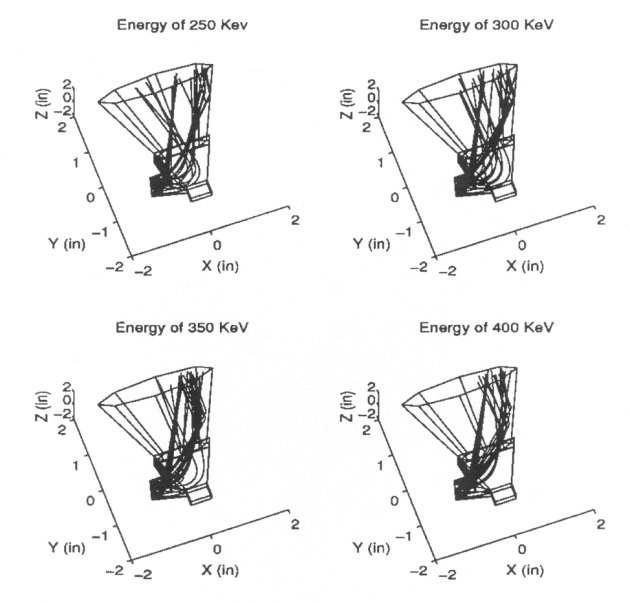Click here for the trajectories for Neubert's backscattering coefficients.